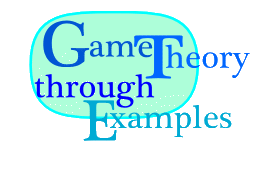MAT109 · Erich Prisner · Franklin College · 2007-2009# Short Version of Chapter 8: Mixed Strategies

Note to the Teacher: ....................
1. A mixed strategy is an assignment of different probabilities to the different pure strategies of a player such that all these probabilities add up to 1. A player plays a given mixed strategy if he or she selects the pure strategies randomly, with these given probabilities belonging to the mixed strategy. Of course, every player which has at least two pure strategies has infinitely many mixed strategies.

2. The payoff of a mixed strategy against pure strategies of the other player is computed as expected value using the corresponding payoffs.

3. Among the best responses to a mixed strategy there is always at least one pure one.

4. Brown's fictitious play process: Assume a game is repeated over and over. In each round, each player selects a best response pure strategy to the observed mixed strategy played by the other player. This process can be simulated in the Excel sheet "Brown.xls".

5. Indifference Theorem: A mixed strategy of Ann is a best response to some (mixed) strategy of Beth if each pure strategy occuring with nonempty probability in Ann's mixed strategy is also a best response to Beth's strategy.

6. von Neumann's Theorem : Every finite two-person zero-sum game has at least one Nash equilibrium in (pure or) mixed strategies. They are the Maximin mixed strategies and all have identical payoffs.

7. Theorem (Julia Robinson) [Robinson 1951]: For 2-player zero-sum games, the mixed strategies obtained converge (get closer and closer) to such a pair of mixed strategies forming a Nash equilibrium.

8. This may also be true for more than 2 players or non-zero-sum game, but it doesn't have to.

9. Nash's Theorem (1950): Every finite game has at least one Nash equilibrium in (pure or) mixed strategies.